Question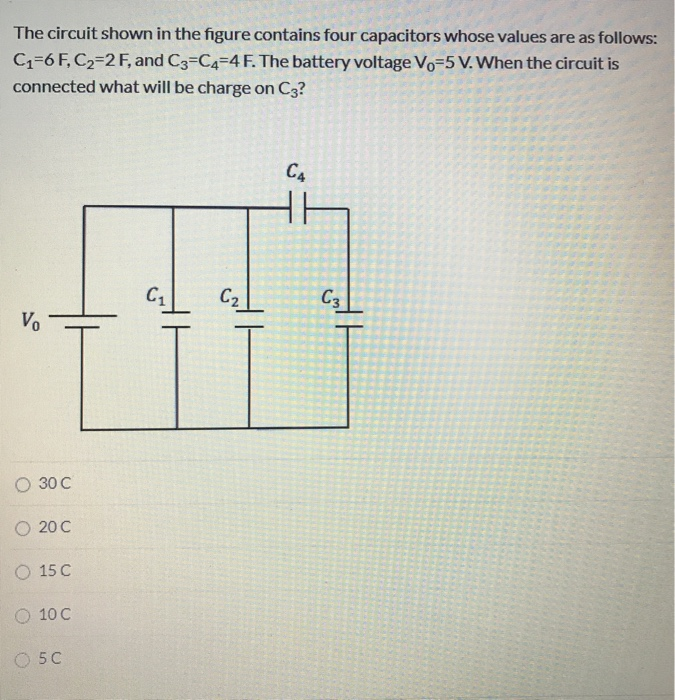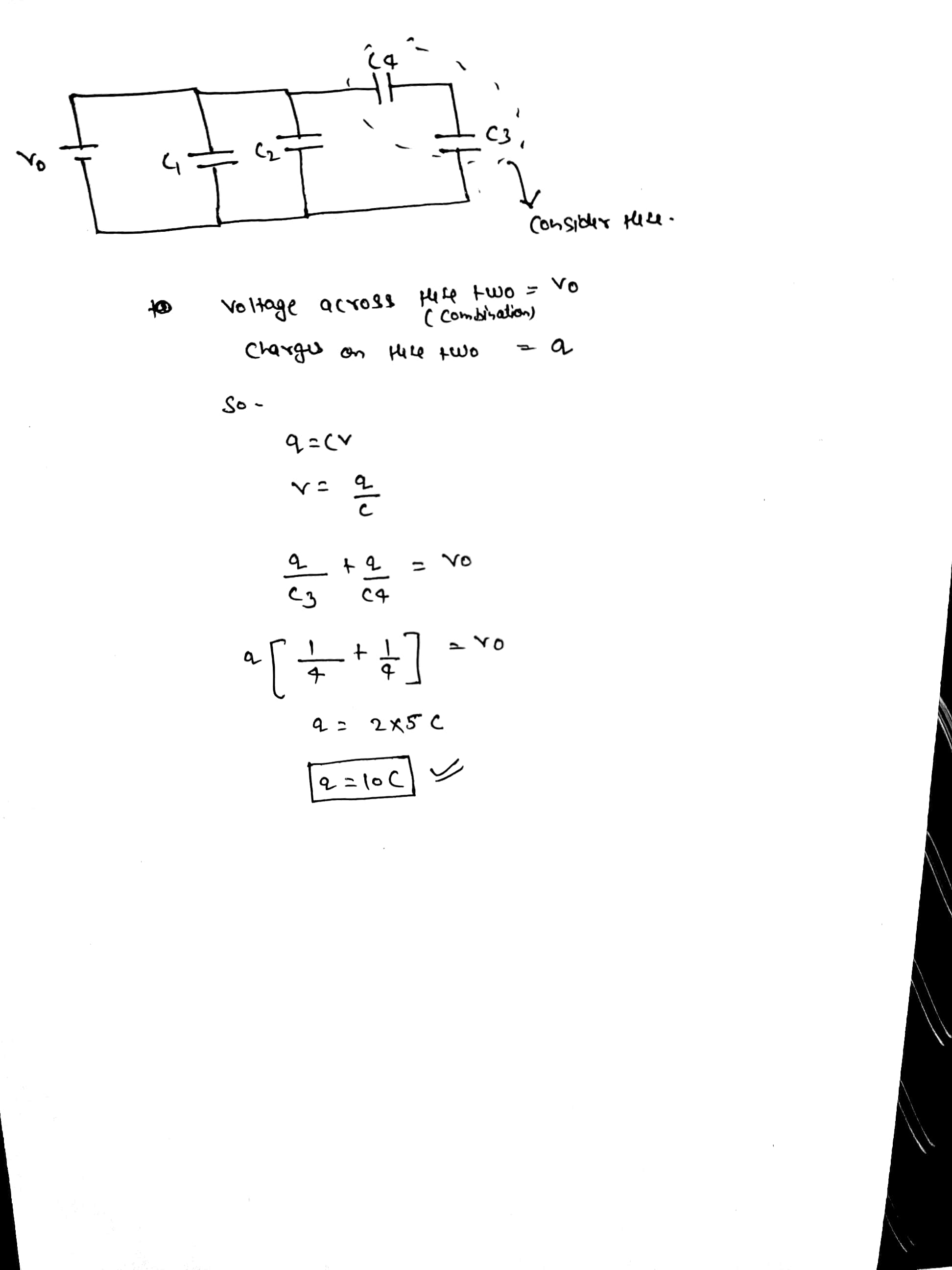#### Earn Coins

Coins can be redeemed for fabulous gifts.

Similar Homework Help Questions
• ### The circuit shown in the figure contains four capacitors whose values are as follows: C1=6 F,...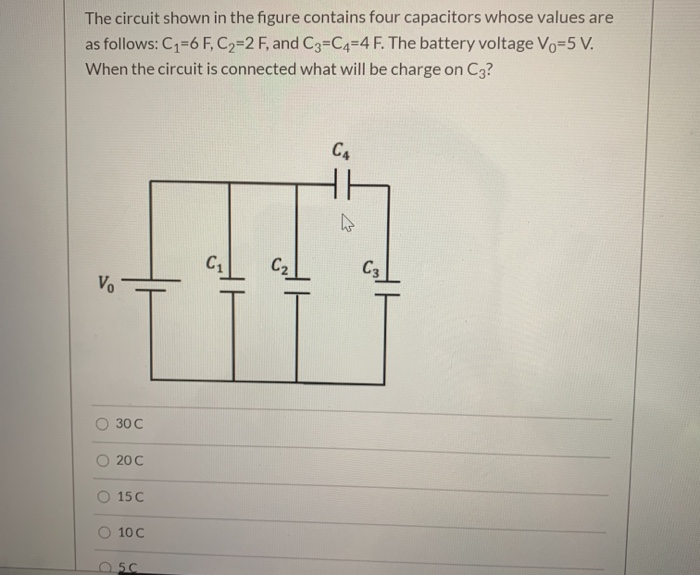The circuit shown in the figure contains four capacitors whose values are as follows: C1=6 F, C2=2 F, and C3=C4=4 F. The battery voltage Vo=5 V. When the circuit is connected what will be charge on C3? CA C1 C2 C3 V. 30C 200 15 C 100 osc

• ### The circuit shown in the figure contains four capacitors whose values are as follows: C1=6 F,...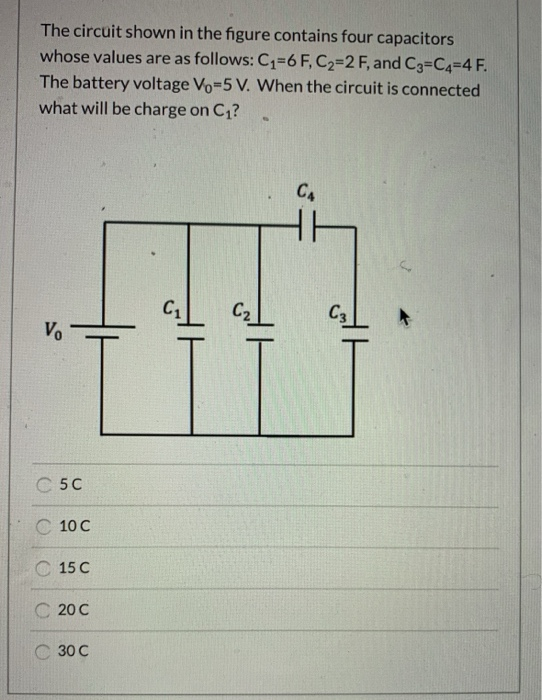The circuit shown in the figure contains four capacitors whose values are as follows: C1=6 F, C2=2 F, and C3=C4=4 F. The battery voltage Vo=5 V. When the circuit is connected what will be charge on C1? CA C2 C3 Vo C5C C100 15 C C20C 30C

• ### The circuit shown in the figure contains four capacitors whose values are as follows: C1=6 F,...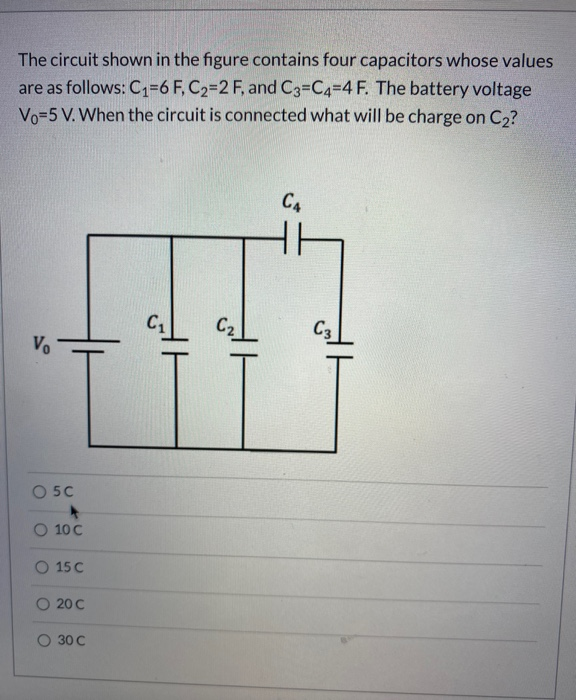The circuit shown in the figure contains four capacitors whose values are as follows: C1=6 F, C2=2 F, and C3=C4=4 F. The battery voltage Vo=5 V. When the circuit is connected what will be charge on C2? C4 C2) V. will alt O 50 O 100 O 15 C O 200 O 30C

• ### The circuit shown in the figure contains four capacitors whose values are as follows: C1=6 F,...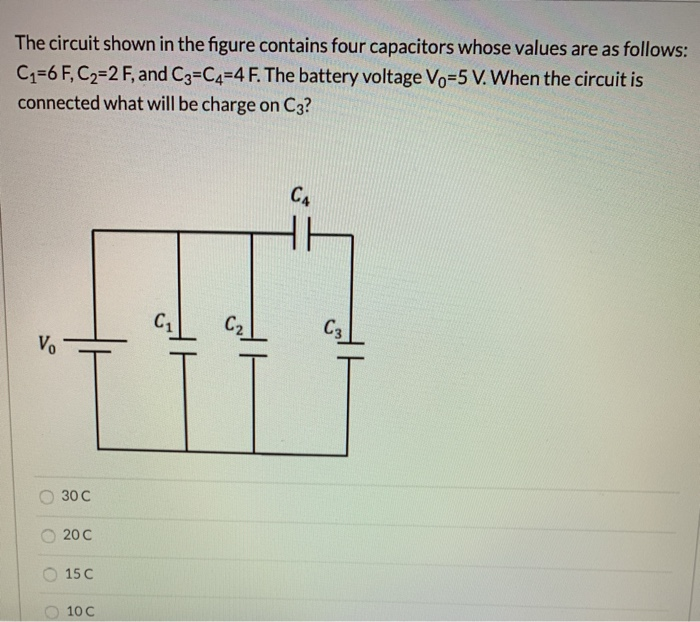The circuit shown in the figure contains four capacitors whose values are as follows: C1=6 F, C2=2 F, and C3=C4=4 F. The battery voltage Vo=5 V. When the circuit is connected what will be charge on C3? CA С2 V. alt 30C 20 C 15 C 10 C

• ### The circuit shown in the figure contains four capacitors whose values are as follows: C2=6 F,...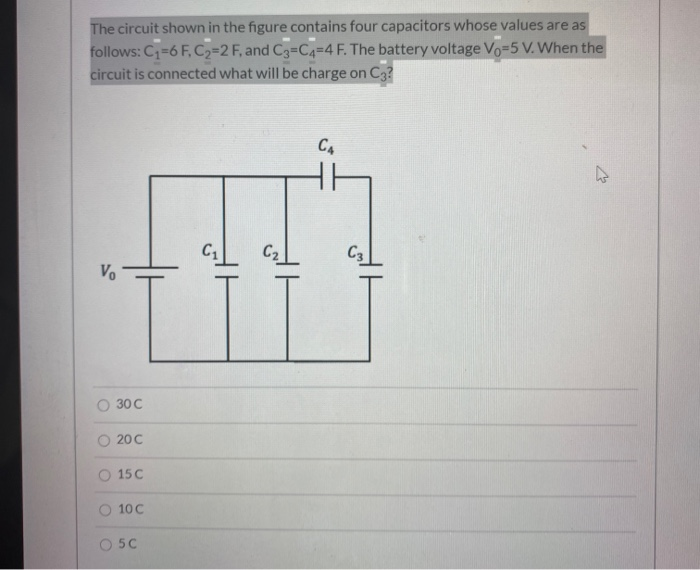The circuit shown in the figure contains four capacitors whose values are as follows: C2=6 F, C2=2 F, and C3=C4=4 F. The battery voltage Vo=5 V. When the circuit is connected what will be charge on C3? CA C1 C2 C3 V. 30C 200 150 100 50

• ### The circuit shown in the figure contains four capacitors whose values are as follows: C1=6 F,...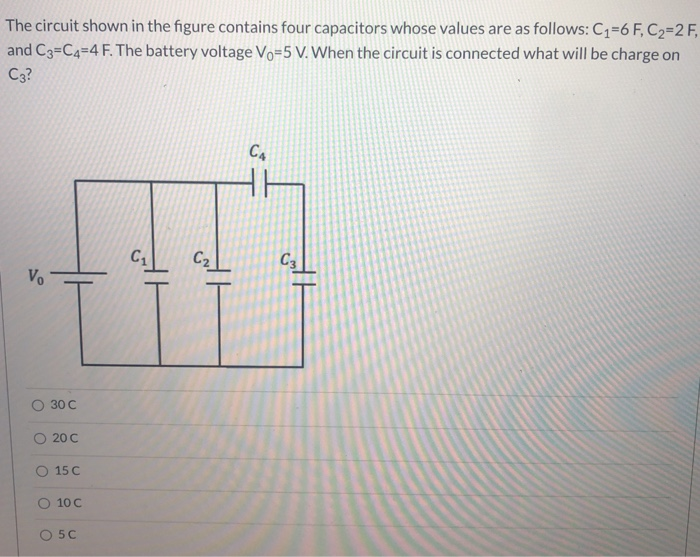The circuit shown in the figure contains four capacitors whose values are as follows: C1=6 F, C2=2 F, and C3=C4=4 F. The battery voltage Vo=5 V. When the circuit is connected what will be charge on C3? CA C1 C2 C3 ܘܢ 30 C O 200 O 15 C O 100 O 5C

• ### The circuit shown in the figure contains four capacitors whose values are as follows: C1=6F, C2=2...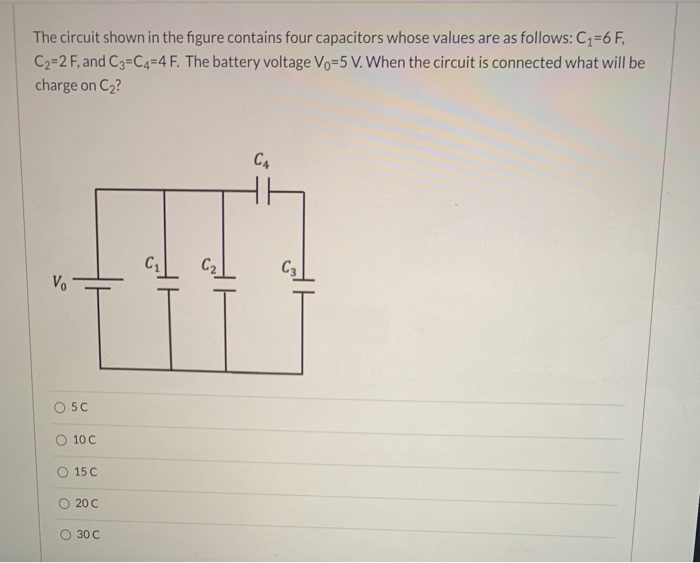The circuit shown in the figure contains four capacitors whose values are as follows: C1=6F, C2=2 F, and C3=C4=4 F. The battery voltage Vo=5 V. When the circuit is connected what will be charge on Cz? CA C C₂ C₂ V. 05C 10 C O 15 C 200 O 30C

• ### The circuit shown in the figure contains four capacitors whose values are as follows: C1=6 F,...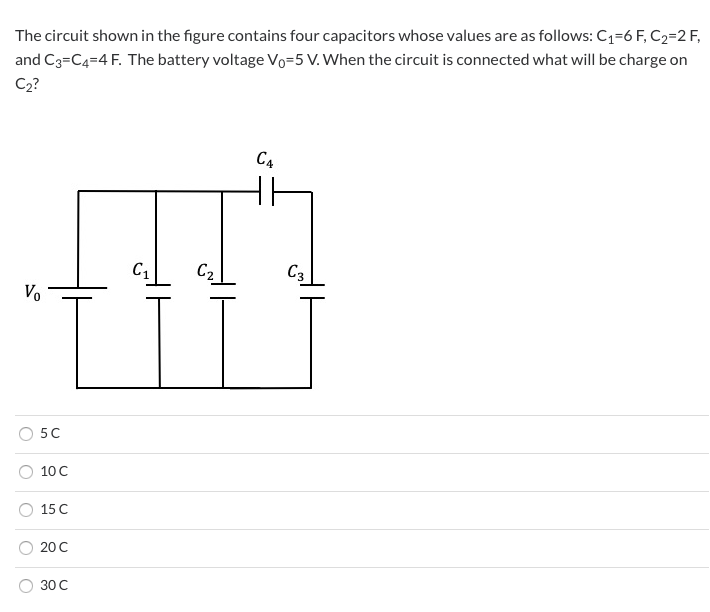The circuit shown in the figure contains four capacitors whose values are as follows: C1=6 F, C2=2 F, and C3=C4=4 F. The battery voltage Vo=5 V. When the circuit is connected what will be charge on C2? C4 C1 C2 C3 Vo 5C 10 C 15 C 200 30 C

• ### The circuit shown in the figure contains four capacitors whose values are as follows: C1=6 F,...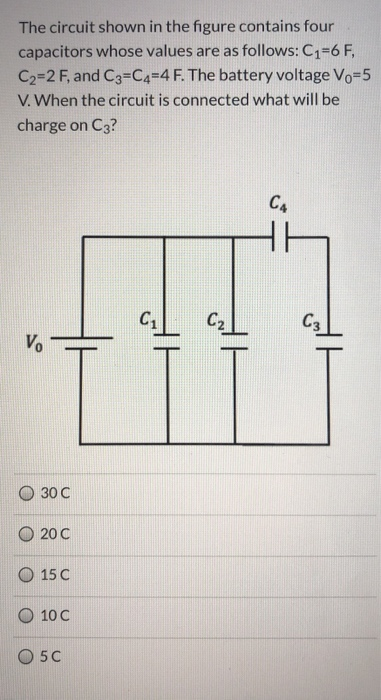The circuit shown in the figure contains four capacitors whose values are as follows: C1=6 F, C2=2 F, and C3=C4=4 F. The battery voltage Vo=5 V. When the circuit is connected what will be charge on C3? CA C₂ C3 V. HH 30 C 200 15 C 10 C O5C

• ### The circuit shown in the figure contains four capacitors whose values are as follows: C1=6 F,...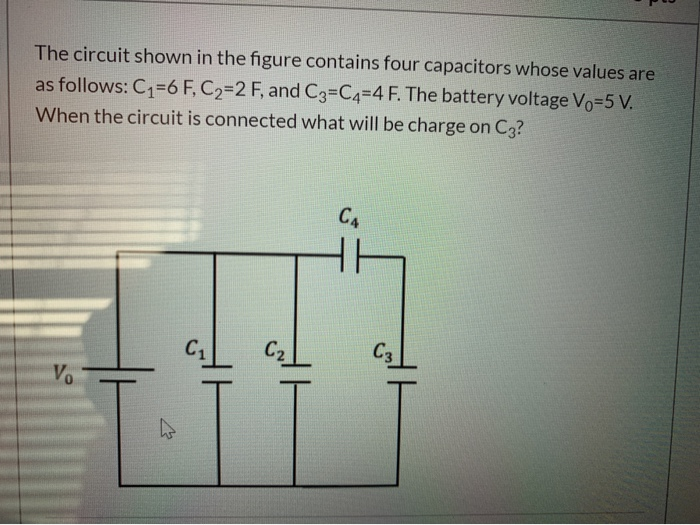The circuit shown in the figure contains four capacitors whose values are as follows: C1=6 F, C2=2 F, and C3=C4=4 F. The battery voltage Vo=5 V. When the circuit is connected what will be charge on C3? CA C₂ C3 V. alt